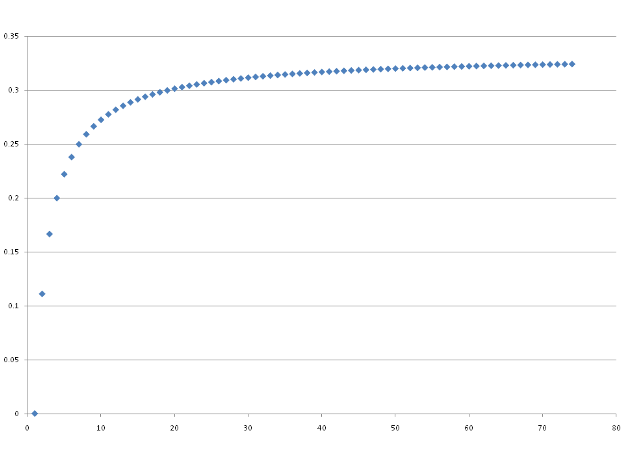#### You may also like### Areas and Ratios

Do you have enough information to work out the area of the shaded quadrilateral?### Napoleon's Hat

Three equilateral triangles ABC, AYX and XZB are drawn with the point X a moveable point on AB. The points P, Q and R are the centres of the three triangles. What can you say about triangle PQR?### Plane to See

P is the midpoint of an edge of a cube and Q divides another edge in the ratio 1 to 4. Find the ratio of the volumes of the two pieces of the cube cut by a plane through PQ and a vertex.

# Eudiometry

##### Age 16 to 18 Challenge Level:

In this problem, consideration of the ideal gas equation is important. At a basic level we know that a mole of substance in gaseous form occupies a volume of roughly 24dm$^3$. The ideal gas equation, $pV = nRT$ has several variables, but at constant temperature and pressure it can be seen that $V = n(\frac{RT}{p})$ where the bracketed term is a constant (R being the ideal gas constant). Thus volume varies directly with number of moles for an ideal gas so consideration of molar changes is sufficient throughout this question.

On combustion of ethane, the balanced equation shows that 2 + 7 = 9 molar units of reactants interact to produce 4 + 6 = 10 molar units of products. As the volume occupied by a mole of gas is considered to be the same regardless of the species involved and that all species are in gaseous form, the volume of the gas must increase proportionally to the increase in moles that has occured.

If you now consider the case for a generic hydrocarbon, we can first analyse the volumetric change for alkanes, which have a generic formula C$_n$H$_{2n + 2}$, and alkenes or cyclic alkanes which have a general formula C$_n$H$_{2n}$. These examples cover the most widely encountered hydrocarbons. A further calculation may be conducted for benzene.

Alkanes

C$_n$H$_{2n + 2}$ + $\frac{3n + 1}{2}$O$_2$ $\rightarrow$ nCO$_2$ + (n + 1)H$_2$O

so in terms of a molar change:

$1 + \frac{3n + 1}{2}\rightarrow 2n + 1$

Proportional increase in molesNumber of carbon atoms

It can be seen that the increase in gaseous volume on combustion is by no means assured for all hydrocarbons. For CH$_4$ for example, there is no increase in the number of moles of gas on combustion. As alkanes get larger the proportional increase in volume increases and tends to 0.33 due to the dominance of $\frac{3n}{2}$ for the left hand side and 2n for the right hand side of the equation at large values of n in determining the number of moles.
$\frac{2n - \frac{3n}{2}}{\frac{3n}{2}}$ = 0.33 (2dp).
Thus large chain alkanes produce a large change in volume as although the percentage change tends to 33%, the actual number of reactant moles increases considerably with the addition of 1.5 molar units for total reactant moles for an increase of a single carbon.
Alkenes
Alkenes show a very similar relationship to that above.
C$_n$H$_{2n}$ + $\frac{3n}{2}$O$_2$ $\rightarrow$ nCO$_2$ + nH$_2$O
In terms of the molar changes:
1 + $\frac{3n}{2}$ $\rightarrow$ 2n
Again, the percentage increase in volume tends to 33% as with alkanes. Yet for each species equivalent in terms of the number of carbons to a certain alkane, the actual increase in moles is slightly greater for the alkane due to the presence of a greater number of hydrogen atoms in the species (and the fact that a single molecule of oxygen is utilised to generate two water molecules). Note also that ethene is the alkene species for which there is no increase in volume on combustion.
Cycloalkanes have the same general formula as alkenes but obviously it is impossible to produce a two membered ring structure.
As a point of interest, benzene has a molecular formula C$_6$H$_6$ and its complete combustion in oxygen is given below.
C$_6$H$_6$ + $\frac{15}{2}$O$_2$ $\rightarrow$ 6CO$_2$ + 3H$_2$O
8.5 moles $\rightarrow$ 9 moles
This corresponds to a marginal increase in volume. Considering the trends for these types of molecules it is clear that the molar increase in volume is greatest for long chain alkanes due to the fact that these a saturated molecules which require a large number of reactant oxygen molecules for combustion.
As we have seen, if the total volume of gas is unchanged by reaction the number of moles on the left and right hand sides should be equivalent. An example of a reaction where the number of moles of gas remains unchanged is:
N$_2$ + O$_2$ $\rightarrow$ 2NO
Can you think of any other examples?